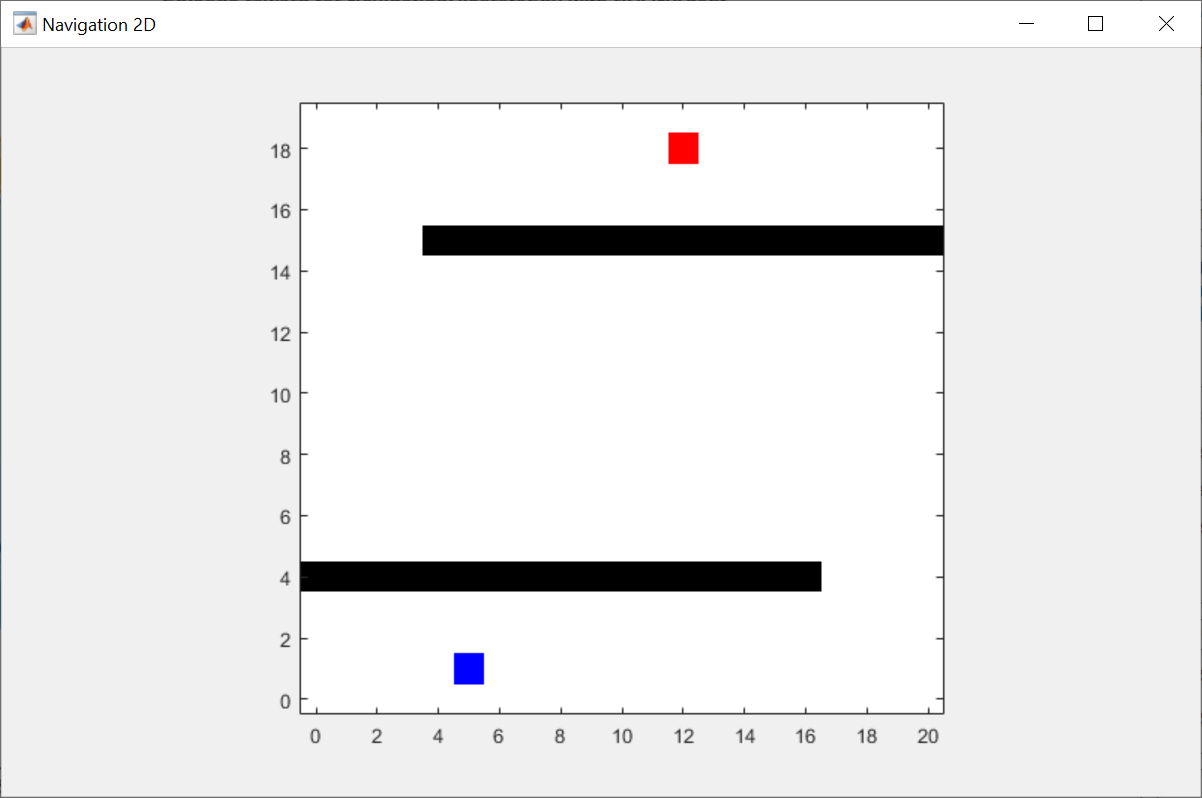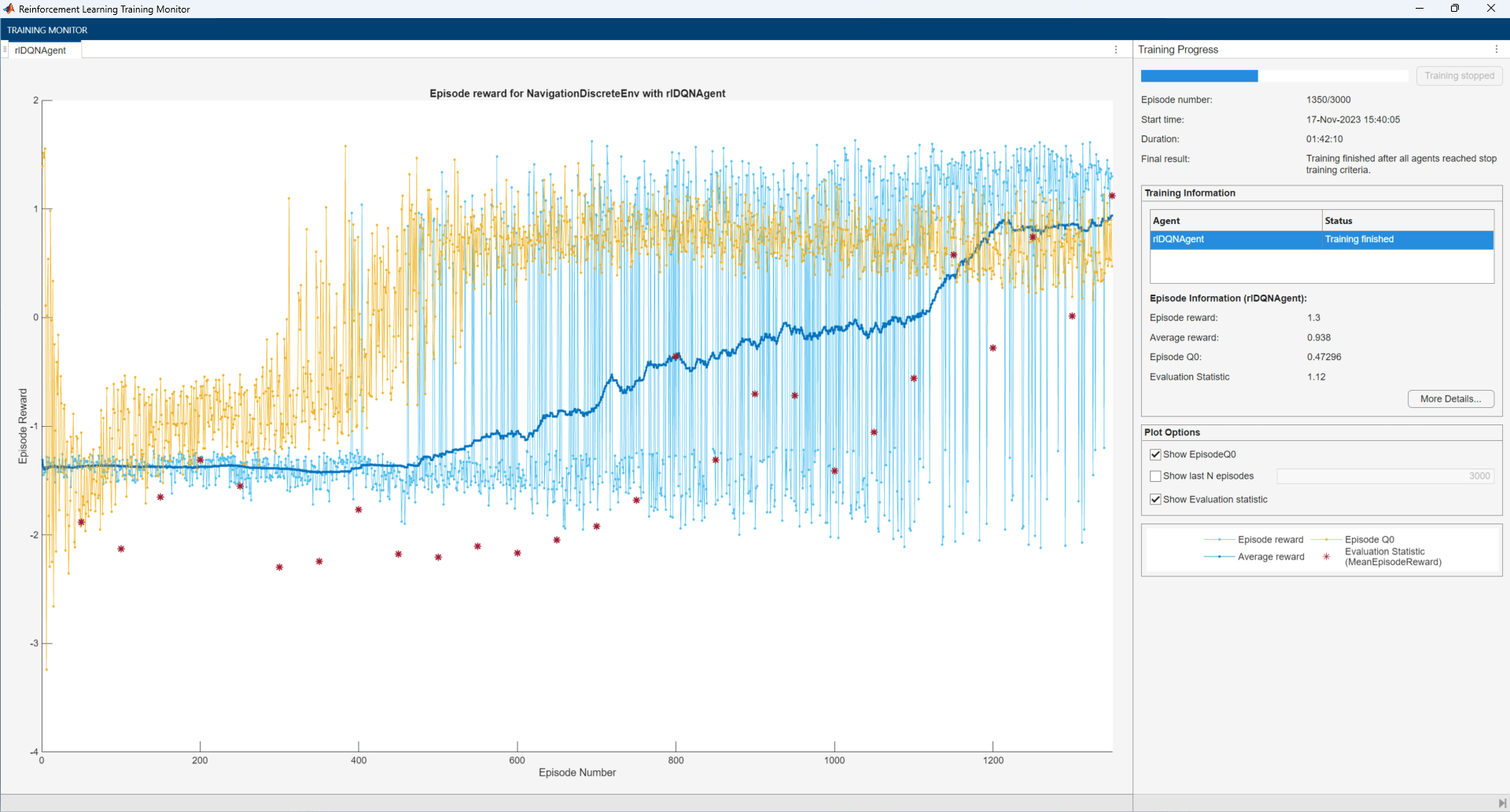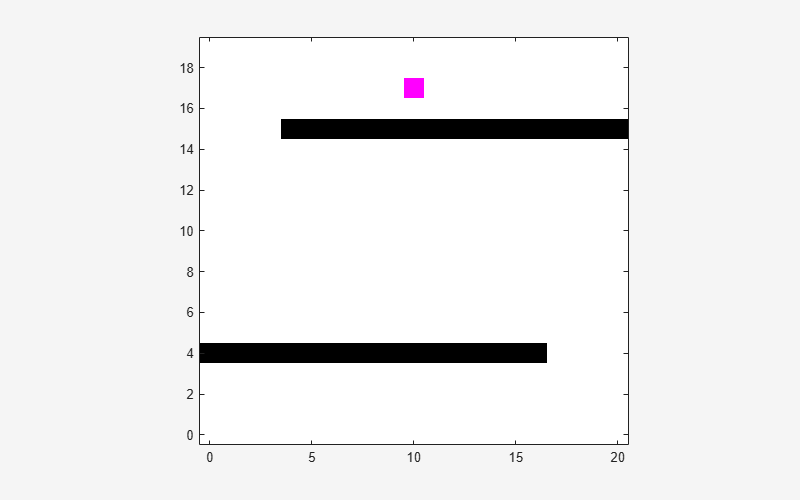# Train DQN Agent Using Hindsight Experience Replay

This example shows how to train a deep Q-learning network (DQN) agent using hindsight experience replay in a discrete navigation problem.

The reinforcement learning environment for this example is a simple discrete 2D navigation problem. The training goal is to make a robot reach the goal state.

The environment is a 20-by-20 grid containing a robot (blue), a goal (red), and obstacles (black).The navigation problem in this example is a goal-conditioned task for which the observation from the environment contains both the goal (navigation target) and the goal measurement (robot position). The observation column vector contains the robot position $\left({\mathit{x}}_{\mathrm{robot}},{\mathit{y}}_{\mathrm{robot}}\right)$ and the navigation target $\left({\mathit{x}}_{\mathrm{goal}},{\mathit{y}}_{\mathrm{goal}}\right)$.

`$\mathit{O}={\left[{\mathit{x}}_{\mathrm{robot}},{\mathit{y}}_{\mathrm{robot}},{\mathit{x}}_{\mathrm{goal}},{\mathit{y}}_{\mathrm{goal}}\right]}^{\mathit{T}}$`

The initial position of the robot and the goal are sampled as follows:

`$\begin{array}{l}{\mathit{x}}_{\mathrm{robot}}~\mathit{U}\left\{0,19\right\}\\ {\mathit{y}}_{\mathrm{robot}}~\mathit{U}\left\{0,3\right\}\\ {\mathit{x}}_{\mathrm{goal}}~\mathit{U}\left\{0,19\right\}\\ {\mathit{y}}_{\mathrm{goal}}~\mathit{U}\left\{16,19\right\}\end{array}$`

where $\mathit{U}\left\{\mathit{a},\mathit{b}\right\}$ is a discrete uniform distribution between $\mathit{a}$ and $\mathit{b}$.

The discrete actions for this environment are defined as follows:

`$\mathit{A}=\left\{\begin{array}{ll}1& {\mathit{y}}_{\mathrm{robot}}={\mathit{y}}_{\mathrm{robot}}+1\text{\hspace{0.17em}\hspace{0.17em}\hspace{0.17em}\hspace{0.17em}}\left(\mathrm{Go}\text{\hspace{0.17em}}\mathrm{up}\right)\\ 2& {\mathit{y}}_{\mathrm{robot}}={\mathit{y}}_{\mathrm{robot}}-1\text{\hspace{0.17em}\hspace{0.17em}\hspace{0.17em}\hspace{0.17em}}\left(\mathrm{Go}\text{\hspace{0.17em}}\mathrm{down}\right)\\ 3& {\mathit{x}}_{\mathrm{robot}}={\mathit{x}}_{\mathrm{robot}}-1\text{\hspace{0.17em}\hspace{0.17em}\hspace{0.17em}\hspace{0.17em}}\left(\mathrm{Go}\text{\hspace{0.17em}}\mathrm{left}\right)\\ 4& {\mathit{x}}_{\mathrm{robot}}={\mathit{x}}_{\mathrm{robot}}+1\text{\hspace{0.17em}\hspace{0.17em}\hspace{0.17em}\hspace{0.17em}}\left(\mathrm{Go}\text{\hspace{0.17em}}\mathrm{right}\right)\end{array}$`

If the action leads to the obstacle location or the map's boundary, the robot doesn't move.

The reward signal is defined as follows:

`$\mathit{R}=\left\{\begin{array}{ll}2& \mathrm{if}\text{\hspace{0.17em}}{\mathit{x}}_{\mathrm{robot}}={\mathit{x}}_{\mathrm{goal}}\text{\hspace{0.17em}}\mathrm{and}\text{\hspace{0.17em}}{\mathit{y}}_{\mathrm{robot}}={\mathit{y}}_{\mathrm{goal}}\\ -0.01-0.01\mathit{I}\left(\mathit{O},{\mathit{O}}^{\prime }\right)& \mathrm{otherwise}\end{array}$`

where ${\mathit{O}}^{\prime }\text{\hspace{0.17em}}$is the next observation, and

`$\mathit{I}\left(\mathit{O},{\mathit{O}}^{\prime }\right)=\left\{\begin{array}{ll}1& \text{\hspace{0.17em}}\mathrm{if}\text{\hspace{0.17em}}\mathrm{the}\text{\hspace{0.17em}}\mathrm{robot}\text{\hspace{0.17em}}{\mathrm{doesn}}^{\prime }\mathit{t}\text{\hspace{0.17em}}\mathrm{move}\text{\hspace{0.17em}}\mathrm{due}\text{\hspace{0.17em}}\mathrm{to}\text{\hspace{0.17em}}\mathrm{obstacles}\text{\hspace{0.17em}}\mathrm{or}\text{\hspace{0.17em}}\mathrm{boundaries}.\\ 0& \mathrm{otherwise}\end{array}$`

Terminal condition is defined as follows:

`$\mathrm{IsDone}=\left\{\begin{array}{ll}1& \mathrm{if}\text{\hspace{0.17em}}{\mathit{x}}_{\mathrm{robot}}={\mathit{x}}_{\mathrm{goal}}\text{\hspace{0.17em}}\mathrm{and}\text{\hspace{0.17em}}{\mathit{y}}_{\mathrm{robot}}={\mathit{y}}_{\mathrm{goal}}\\ 0& \mathrm{otherwise}\end{array}$`

### Create Environment Interface

Fix the random generator seed for reproducibility.

`rng(1)`

Create a discrete navigation environment. `NavigationDiscreteEnv.m` is located in this example folder.

`env = NavigationDiscreteEnv;`

### Create DQN Agent

For a given observation and action, a DQN agent estimates the value of the policy (the cumulative discounted long-term reward) using a parametrized Q-value function critic.

Create a default DQN agent from the environment specifications. For more information `on` DQN agents, see `rlDQNAgent`.

```obsInfo = getObservationInfo(env); actInfo = getActionInfo(env); agent = rlDQNAgent(obsInfo,actInfo);```

Specify the DQN agent options, including training options for the critic. Alternatively, you can use `rlDQNAgentOptions` object.

```agent.AgentOptions.EpsilonGreedyExploration.EpsilonDecay = 1e-5; agent.AgentOptions.EpsilonGreedyExploration.Epsilon = 0.2; agent.AgentOptions.MiniBatchSize = 128; agent.AgentOptions.CriticOptimizerOptions.LearnRate = 5e-4; agent.AgentOptions.CriticOptimizerOptions.GradientThreshold = 1;```

### Construct Hindsight Replay Memory

The DQN agent is unable to efficiently learn a good policy with a default replay memory because of the sparse rewards. You can improve the sample efficiency by using a hindsight replay memory for a goal-conditioned task with sparse rewards. The hindsight experience replay augments the acquired experiences by replacing the goal with the goal measurement so that agent can use the data that reaches the replaced goal. Thus, the agent can be trained with meaningful rewards even if the agent does not reach the goal.

To use a hindsight replay memory, set `ExperienceBuffer` of the agent to `rlHindsightReplayMemory`. You need to specify the following.

• A reward function: The reward function, `myNavigationGoalRewardFcn`, computes the true reward given observation, action, and next observation.

• An is-done function: The is-done function, `myNavigationGoalIsDoneFcn`, computes the true is-done signal given observation, action, and the next observation.

• Goal condition information: `goalConditionInfo` specifies the channel and indices of goal measurements and goals. In this example, there is only one observation channel; the first and second observation elements correspond to the goal measurements (the robot position), and the third and fourth observation elements correspond to the goal position.

• Experience buffer length (optional): This examples uses 1e5.

`myNavigationGoalRewardFcn.m` and `myNavigationGoalIsDoneFcn.m` are located in this example folder. For more information, type `help rlHindsightReplayMemory`.

To use a standard replay memory, set `useHER `to `false`.

```useHER = true; if useHER rewardFcn = @myNavigationGoalRewardFcn; isDoneFcn = @myNavigationGoalIsDoneFcn; % observation is [robot_x, robot_y, goal_x, goal_y]' % goal measurement: channel --- 1, indices --- 1,2 % goal: channel --- 1, indices --- 3,4 goalConditionInfo = {{1,[1,2], 1, [3,4]}}; bufferLength = 1e5; agent.ExperienceBuffer = rlHindsightReplayMemory(obsInfo,actInfo,... rewardFcn,isDoneFcn,goalConditionInfo,bufferLength); else agent.AgentOptions.ExperienceBufferLength = 1e5; end```

### Train Agent

To train the agent, first specify the training options. For this example, use the following options:

• Run one training session containing 3000 episodes, with each episode lasting at most 120 time steps.

• Display the training progress in the Episode Manager dialog box (set the `Plots` option) and disable the command line display (set the `Verbose` option to `false`).

• Set the Plots options to "training-progress"

For more information, see `rlTrainingOptions`.

```maxEpisodes = 3000; maxStepsPerEpisode = 120; trainOpts = rlTrainingOptions(... MaxEpisodes=maxEpisodes, ... MaxStepsPerEpisode=maxStepsPerEpisode, ... Verbose=false, ... ScoreAveragingWindowLength=100,... Plots="training-progress",... StopTrainingCriteria="EpisodeCount",... StopTrainingValue=maxEpisodes);```

You can visualize the environment by using the `plot` function during training or simulation.

`plot(env)`Train the agent using the `train` function. Training this agent is a computationally intensive process. To save time while running this example, load a pretrained agent by setting `doTraining` to `false`. To train the agent yourself, set `doTraining` to `true`.

```doTraining = false; if doTraining % Train the agent. trainingStats = train(agent,env,trainOpts); else % Load the pretrained agent for the example. load("DiscreteNavigationDQNAgent.mat","agent") end```

The following figure shows a snapshot of training progress.### Simulate DQN Agent

Since the reset function randomizes the reference values, fix the random generator seed to ensure simulation reproducibility.

`rng(2)`

To validate the performance of the trained agent, simulate it within the navigation environment. For more information on agent simulation, see `rlSimulationOptions` and `sim`.

```simOptions = rlSimulationOptions(... NumSimulations=1,... MaxSteps=maxStepsPerEpisode); simResults = sim(env,agent,simOptions);```The trained agent successfully reached the goal.

### Reference

Andrychowicz, Marcin, Filip Wolski, Alex Ray, Jonas Schneider, Rachel Fong, Peter Welinder, Bob McGrew, Josh Tobin, Pieter Abbeel, and Wojciech Zaremba. "Hindsight experience replay." Advances in neural information processing systems 30 (2017).